# KSEEB SSLC Class 10 Maths Solutions Chapter 5 Areas Related to Circles Ex 5.2

In this chapter, we provide KSEEB SSLC Class 10 Maths Solutions Chapter 5 Areas Related to Circles Ex 5.2 for English medium students, Which will very helpful for every student in their exams. Students can download the latest KSEEB SSLC Class 10 Maths Solutions Chapter 5 Areas Related to Circles Ex 5.2 pdf, free KSEEB SSLC Class 10 Maths Solutions Chapter 5 Areas Related to Circles Ex 5.2 book pdf download. Now you will get step by step solution to each question.

### Karnataka State Syllabus Class 10 Maths SolutionsChapter 5 Areas Related to Circles Ex 5.2

{Unless stated otherwise, use π=227}

Question 1.
Find the area of a sector of a circle with radius 6 cm if angle of the sector is 60°.
Solution:
Radius of a sector of a circle, r = 6 cm.
Angle of a sector of a circle, θ = 60°
Area of a sector of a circle = ?
Area of a sector of a circle =θ360×πr2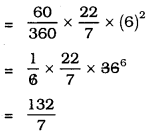= 18.8 sq.cm.

Question 2.
Find the area of a quadrant of a circle whose circumference is 22 cm.
Solution:
Circumference of a circle, 2πr = 22 cm.
Area of a quadrant of a circle = ?
2πr = 22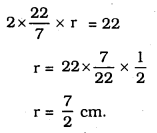∴ Area of a quadrant of a circle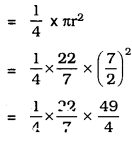=778
= 9.62 sq. cm.

Question 3.
The length of the minute hand of a clock is 14 cm. Find the area swept by the minute hand in 5 minutes.
Solution:
Length of the minute hand of a clock, r = 14 cm.
Area swept by the minute hand in 5 minutes =360×560
= 30°
Angle of segment of a circle, 0 = 30°
∴ Area swept by the minute hand in 5 minute,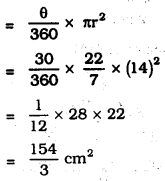Question 4.
A chord of a circle of radius 10 cm subtends a right angle at the centre. Find the area of the corresponding:
(i) minor segment, (ii) major sector. (Use π = 3.14)
Solution:
Radius of the circle, r = 10 cm.
Angle at the centre of circle, θ = 90°
1) Area of minor segment = ?
2) Area of Major segment = ?
(i) Area of minor segment OAPB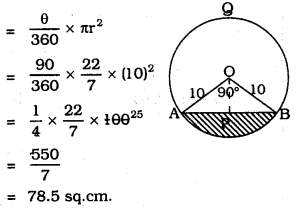ii) Area of Minor Segment, APB :
= Area of OAPB – Area of AOAB
=78.5cm2⋅−12×OA×OB
=78.5−12×10×10
= 78.5 – 50
= 28.5 sq. cm.

(iii) Area of the Major Segment (AQBO):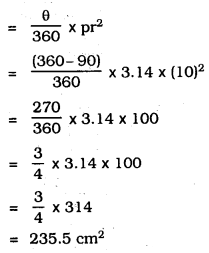Question 5.
In a circle of radius 21 cm, an arc subtends an angle of 60° at the Centre. Find:
(i) the length of the arc,
(ii) area of the sector formed by the arc,
(iii) area of the segment formed by the corresponding chord.
Solution:
Radius of the circle, r = 21 cm.
Angle at the centre of the circle, θ = 60°
1) the length of the arc?
2) Area of the sector formed by the arc?
3) Area of the segment.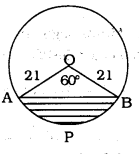1) The length of arc OAPB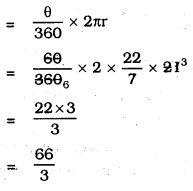= 22 cm

2) Area of the sector OAPB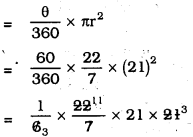= 231 sq. cm.

3) Area of the segment APB:
= Area of the sector OAPB – Area of the equilateral ∆OAB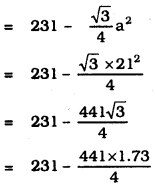= 231 – 190.7
= 40.3 sq. cm

Question 6.
A chord of a circle of radius 15 cm subtends an angle of 60° at the centre. Find the areas of the corresponding minor and major segments of the circle.
(Use π = 3.14 and 3–√=1.73).
Solution:
Radius of circle, r = 15 cm.
Angle at the centre, θ = 60°
1) Area of the minor segment =?
2) Area of the Major segment =?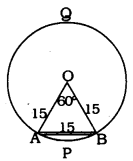(i) Area of Minor segment, APB :
= Area of sector OAPB – Area of ∆OAB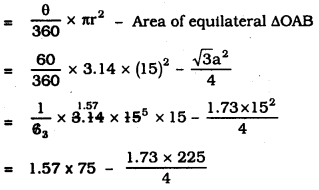= 117.75 sq. cm. – 97.31
= 20.44 sq. cm.

ii) Area of the Major segment:
= Area of Circle – Area of a segment of circle OAPB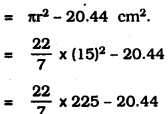= 3.14 × 225 – 20.44
= 707.14 – 20.44
= 686.06 cm2.

Question 7.
A chord of a circle of radius 12 cm subtends an angle of 120° at the centre. Find the area of the corresponding segment of the circle.
(Use π = 3.14 and 3–√=1.73).
Solution:
Radius of the circle, r = 12 cm.
Angle at the centre, θ = 120°
Area of segment APB =?
(i) Area of sector OAPB: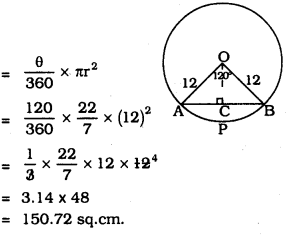(ii) Area of segment APB: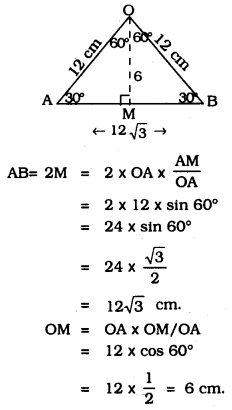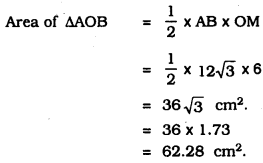∴ Area of segment APB:
= Area of sector OAPB – Area of ∆AOB
= 150.72 – 62.68
= 88.44 sq. cm.

Question 8.
A horse is tied to a peg at one corner of a square-shaped grass field of side 15 m by means of a 5 m. long rope (see Figure). Find
i) the area of that part of the field in which the horse can graze.
ii) the increase in the grazing area if the rope were 10 m long instead of 5 m. (use π = 3.14)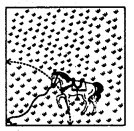Solution:
Length of the rope, r = 5 m, θ = 90°
Area of segment =?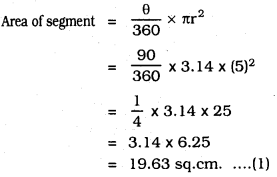(ii) Length of the rope, r = 10 cm, θ = 90°
Area of segment =?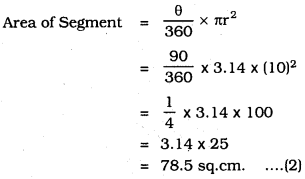∴ Area of the field which is increased in the grazing
= Equation (2) – Equation (1)
= 78.5 – 19.63
= 58.87 sq. cm.

Question 9.
A brooch is made with silver wire in the form of a circle with a diameter of 35 mm. The wire is also used in making 5 diameters which divide the circle into 10 equal sectors as shown in the Figure. Find
i) the total length of the silver wire required,
ii) the area of each sector of the brooch.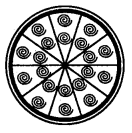Solution:
Diameter, d = 35mm θ = 360∘10 = 36°
(i) Length of the silver wire required :
Length of the wire used in making 5 diameter + Circumference of Silver, wire required.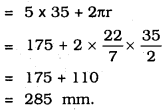(ii) Area of each sector of the brooch = ?
Diameter of the brooch, d = 35mm
Angle at the centre, θ = 360∘10 = 36°
The Area of each sector of the Brooch: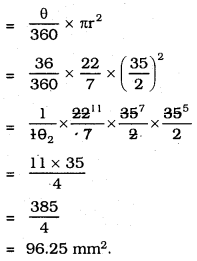Question 10.
An umbrella has 8 ribs which are equally spaced (see the figure). Assuming the umbrella to be a flat circle of radius 45 cm, find the area between the two consecutive ribs of the umbrella.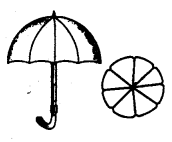Solution:
Radius of circle, r = 45 cm.
Angle at the centre, θ = 360∘8 = 45°
Area between the two consecutive ribs of the umbrella =?
Area of each segment of the circle,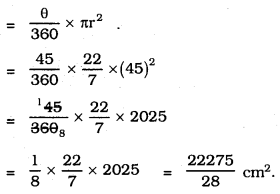Question 11.
A car has two wipers which do not overlap. Each wiper has a blade of length 25 cm sweeping through an angle of 115°. Find the total area cleaned at each sweep of the blades.
Solution:
Radius of the wiper of the car, r = 25 cm.
Angle at the centre, θ = 115°
Area of the sector =?
Area of the blade when it is swept once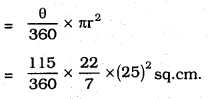Area of two blades when swept: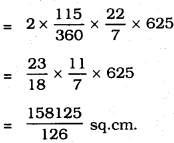Question 12.
To warn ships for underwater rocks, a lighthouse spreads a red coloured light over a sector of angle 80° to a distance of 16.5 km. Find the area of the sea over which the ships are warned. (use π = 3.14)
Solution:
Radius, r = 16.5 km. = 332 km.
Angle, θ = 80°
Area of the Sector =?
Area of the sea over which the ships are warned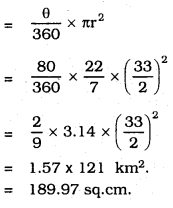Question 13.
A round table cover has six equal designs as shown in Figure. If the radius of the cover is 28 cm, find the cost of making the designs at the rate of Rs. 0.35 per cm2.
(Use 3–√= = 1.7)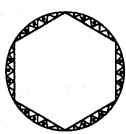Solution:
Each angle at the centre,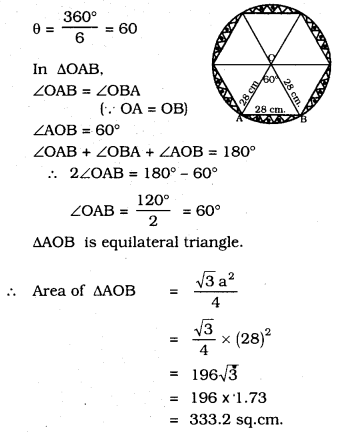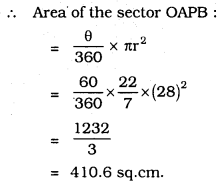Area of segment APB design 1 :
= Area of OAPB – Area of ∆AOB
= 410.6 – 333.2
= 77.46 sq.cm.
∴ Total are of SIX designs = 6 × 77.46
= 464.8 sq.cm.
Cost of designing for 1 sq.cm, is Rs. 0.35
∴ Cost of designing for 464.8 sq.cm ………….
= 464.8 × 0.35
= Rs. 162.68

Question 14.
Tick the correct answer in the following:
Area of a sector of angle p (in degrees) of a circle with radius R is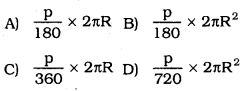Solution:
D) p720 × 2πR2

All Chapter KSEEB Solutions For Class 10 Maths

—————————————————————————–

All Subject KSEEB Solutions For Class 10

*************************************************

I think you got complete solutions for this chapter. If You have any queries regarding this chapter, please comment on the below section our subject teacher will answer you. We tried our best to give complete solutions so you got good marks in your exam.

If these solutions have helped you, you can also share kseebsolutionsfor.com to your friends.

Best of Luck!!